cancel
Showing results for
Did you mean: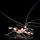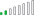Resolver II

## How to create a Slicer based on a range of Calculated Measure

Hi ,  like a slicer that has a range of value, where upon selcting, it will pick a Measure that falls within the range. I tried going thru many examples in the internet but have not come a cross one that address my challenge.

Imaging I have 2 tables (i) Country GDP (ii) Country Defence spending .. I create a measure of DefenceSpending / Country GDP = X%

Using the attached file, the end result if to be able to show the following when I select the slicer

What I am looking for is to select the slicer below and PowerBI table return only Country with measure within the selected range

I have created a new table (without relationship), with the below 4 scenarios BUT i dont know how to make the slicer full the data I wanted

SLICER
0% < 1.0% This should select Myamar, Malaysia, Pakistan, Brunei, Korea
1.0% < 2.0% This should select Philippine, Nepal, Bangladesh, Vietnam, New Zealand
2.0% < 3.0% This should select Sri Lanka, Japan, HongKong
3% or greater This should select Singapore, Thailand, China Taiwan, Indonesia & Australia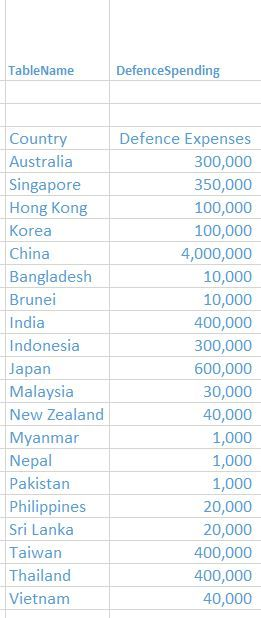2 ACCEPTED SOLUTIONS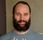Super User

[filterMeasure] is only meant to do just that, it filters the data based on your slicer selection, and returns the number of rows which satisfy the current filter context.

Since you have not posted any data or pbix-file, I have scraped together some data which resembles your data here:
GDP vs Military expenditure

Since I don't know how your data look like, you might have to consider the relationship between your tables. And also possibly count the 'Military expenditure'-table instead of the GDP-tableResolver II

This is brillaint,, i replicatre what you did and it works  ,,,,,, so great ,,, i see how u use visual filter, count row to amke this work...

@sturlaws wrote:

[filterMeasure] is only meant to do just that, it filters the data based on your slicer selection, and returns the number of rows which satisfy the current filter context.

Since you have not posted any data or pbix-file, I have scraped together some data which resembles your data here:
GDP vs Military expenditure

Since I don't know how your data look like, you might have to consider the relationship between your tables. And also possibly count the 'Military expenditure'-table instead of the GDP-table

16 REPLIES 16Helper II

@sturlaws  found the problem, I didnt put the filter to visuals I want to slice... worth to mention here that we need to filter the related visuals to call out the filterMeasure ----> filterMeasure is greater than 0, after that everything works.

Thanks for this excellent sample, you saved my day 🙂Super User

Hi,

create a slicer like this:

```filterMeasure =
SWITCH (
TRUE ();
SELECTEDVALUE ( Intervals[Interval] ) = "0% < 1.0%"; CALCULATE (
COUNTROWS ( GDP );
FILTER ( GDP; [Defence vs GDP] >= 0 && [Defence vs GDP] < 1 )
);
SELECTEDVALUE ( Intervals[Interval] ) = "1.0% < 2.0%"; CALCULATE (
COUNTROWS ( GDP );
FILTER ( GDP; [Defence vs GDP] >= 1 && [Defence vs GDP] < 2 )
);
SELECTEDVALUE ( Intervals[Interval] ) = "2.0% < 3.0%"; CALCULATE (
COUNTROWS ( GDP );
FILTER ( GDP; [Defence vs GDP] >= 2 && [Defence vs GDP] < 3 )
);
SELECTEDVALUE ( Intervals[Interval] ) = "3.0% <"; CALCULATE ( COUNTROWS ( GDP ); FILTER ( GDP; [Defence vs GDP] >= 3 ) );
0
)```

and add this measure to the filter part of you visual

cheers,
SResolver II

I have tried this on my project (which have same logic).. However I got an error .. Can you help me identify and resolve the error I am getting at the bottom "calculate function is required".. Many thinks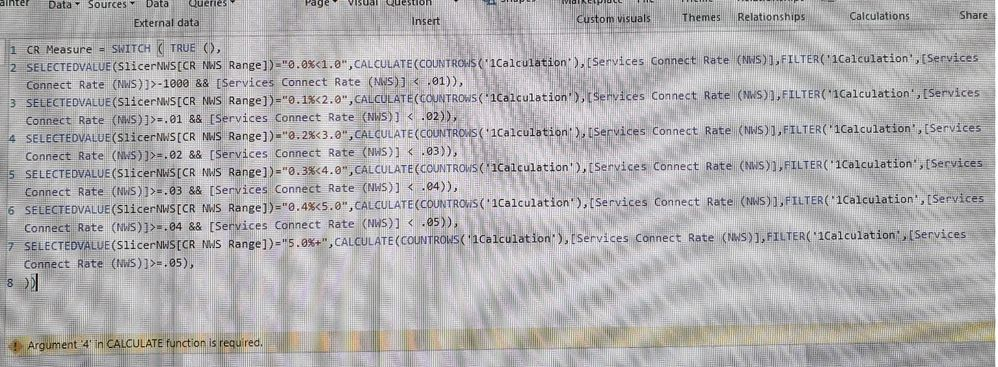Super User

your calculate statements looks like this:

```CALCULATE (
COUNTROWS ( '1Calculation' ),
[Services Connect Rate (NWS)],
FILTER (
'1Calculation',
[Services Connect Rate (NWS)] > -1000
&& [Services Connect Rate (NWS)] < 0.01
)
) ```

After the COUNTROWS-function, you reference [Services Connect Rate (NWS)] which makes no sense. What are you trying to achive with this reference?

Next time, could post the code instead of a snapshot? It makes helping you much easier if I don't have to decipher a unclear image first.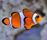Regular Visitor

Start off with an existing Measure I created some time ago  which will return a XX%

Services Connect Rate (NWS) = DIVIDE([DBF+UU+U (NWS)],[DBF(ATU)])

With your help, I am hoping to create the Slicer Measure using your guide

CR Measure = SWITCH ( TRUE (),
SELECTEDVALUE(SlicerNWS[CR NWS Range])="0.0%<1.0",CALCULATE(COUNTROWS('1Calculation'),[Services Connect Rate (NWS)],FILTER('1Calculation',[Services Connect Rate (NWS)]>-1000 && [Services Connect Rate (NWS)] < .01)),
SELECTEDVALUE(SlicerNWS[CR NWS Range])="0.1%<2.0",CALCULATE(COUNTROWS('1Calculation'),[Services Connect Rate (NWS)],FILTER('1Calculation',[Services Connect Rate (NWS)]>=.01 && [Services Connect Rate (NWS)] < .02)),
SELECTEDVALUE(SlicerNWS[CR NWS Range])="0.2%<3.0",CALCULATE(COUNTROWS('1Calculation'),[Services Connect Rate (NWS)],FILTER('1Calculation',[Services Connect Rate (NWS)]>=.02 && [Services Connect Rate (NWS)] < .03)),
SELECTEDVALUE(SlicerNWS[CR NWS Range])="0.3%<4.0",CALCULATE(COUNTROWS('1Calculation'),[Services Connect Rate (NWS)],FILTER('1Calculation',[Services Connect Rate (NWS)]>=.03 && [Services Connect Rate (NWS)] < .04)),
SELECTEDVALUE(SlicerNWS[CR NWS Range])="0.4%<5.0",CALCULATE(COUNTROWS('1Calculation'),[Services Connect Rate (NWS)],FILTER('1Calculation',[Services Connect Rate (NWS)]>=.04 && [Services Connect Rate (NWS)] < .05)),
SELECTEDVALUE(SlicerNWS[CR NWS Range])="5.0%+",CALCULATE(COUNTROWS('1Calculation'),[Services Connect Rate (NWS)],FILTER('1Calculation',[Services Connect Rate (NWS)]>=.05),Regular Visitor

"After the COUNTROWS-function, you reference [Services Connect Rate (NWS)] which makes no sense. What are you trying to achive with this reference?"

Reply, If I select from the slicer, "1.0%<2.0", I want the Table to show the attach Cicrle in BLUEedSuper User

The calculate-function takes these arguments:

`CALCULATE(<expression>,<filter1>,<filter2>…)`

and the way you have written you code you are using the measure [Services Connect Rate (NWS)] as a filter. But a measure by itself like this is not a valid filter argument. Try writting your code like this:

```SELECTEDVALUE ( SlicerNWS[CR NWS Range] ) = "0.0%<1.0", CALCULATE (
COUNTROWS ( '1Calculation' ),
FILTER (
'1Calculation',
[Services Connect Rate (NWS)] > -1000
&& [Services Connect Rate (NWS)] < .01
)
)```Resolver II

will try in morning

by the way, what is the role of the countrow is making this dAX work

`COUNTROWS ( '1Calculation' ),`Super User
The first argument to calculate is a tabel. When using the calculate function it changes the context of how that table is filtered. Countrows counts the rows of the table after it has been filtered. But it not just the filter-function that filters the table, slicers and axis in a report also adds to how the table is filtered.

I used countrows since i don't know how your data looks like. You could use distinct(table[customer id]) instead, or sum(table[sales])Regular Visitor

Hi I still get an error after using this DAX

CR Measure = SWITCH ( TRUE (),
SELECTEDVALUE(SlicerNWS[CR NWS Range])="0.0%<1.0",CALCULATE(COUNTROWS('1Calculation'),FILTER('1Calculation',[Services Connect Rate (NWS)]>-1000 && [Services Connect Rate (NWS)] < .01)),
SELECTEDVALUE(SlicerNWS[CR NWS Range])="0.1%<2.0",CALCULATE(COUNTROWS('1Calculation'),FILTER('1Calculation',[Services Connect Rate (NWS)]>=.01 && [Services Connect Rate (NWS)] < .02)),
SELECTEDVALUE(SlicerNWS[CR NWS Range])="0.2%<3.0",CALCULATE(COUNTROWS('1Calculation'),FILTER('1Calculation',[Services Connect Rate (NWS)]>=.02 && [Services Connect Rate (NWS)] < .03)),
SELECTEDVALUE(SlicerNWS[CR NWS Range])="0.3%<4.0",CALCULATE(COUNTROWS('1Calculation'),FILTER('1Calculation',[Services Connect Rate (NWS)]>=.03 && [Services Connect Rate (NWS)] < .04)),
SELECTEDVALUE(SlicerNWS[CR NWS Range])="0.4%<5.0",CALCULATE(COUNTROWS('1Calculation'),FILTER('1Calculation',[Services Connect Rate (NWS)]>=.04 && [Services Connect Rate (NWS)] < .05)),
SELECTEDVALUE(SlicerNWS[CR NWS Range])="5.0%+",CALCULATE(COUNTROWS('1Calculation'),FILTER('1Calculation',[Services Connect Rate (NWS)]>=.05),
0))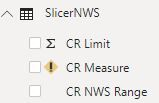Super User

I think you might have misplaced a paranthesis. Your last case statment and else-value looks like this

```SELECTEDVALUE ( SlicerNWS[CR NWS Range] ) = "5.0%+", CALCULATE (
COUNTROWS ( '1Calculation' ),
FILTER ( '1Calculation', [Services Connect Rate (NWS)] >= 0.05 ),
0
))```

when it should have been like this

```SELECTEDVALUE ( SlicerNWS[CR NWS Range] ) = "5.0%+", CALCULATE (
COUNTROWS ( '1Calculation' ),
FILTER ( '1Calculation', [Services Connect Rate (NWS)] >= 0.05 )
),
0
)```Resolver II

Formula seem acceptable, however the look is what i am hoping for.. a web version is publish.. I am using GDP vs Defense spend example instead... https://app.powerbi.com/view?r=eyJrIjoiNzc5YTU3MjUtMjY0OS00NGEzLWIxYzEtOTNlYjA0ZmVkMTQ2IiwidCI6IjdlN...

i the screen shot, the filter is selecting the correct country in the chart BUT, the value incorrectly showing 100.00%

DAX

FilterMeasure =
SWITCH (
TRUE (),
SELECTEDVALUE ('what we want to achieve'[SLICER]) = "0.0%<1.0%", CALCULATE (COUNTROWS (GDP), FILTER ( GDP,[Defense%] >= 0 && [Defense%] < 0.01 )),
SELECTEDVALUE ('what we want to achieve'[SLICER]) = "1.0%<2.0%", CALCULATE (COUNTROWS (GDP), FILTER ( GDP,[Defense%] >= 0.01 && [Defense%] < 0.02 )),
SELECTEDVALUE ('what we want to achieve'[SLICER]) = "2.0%<3.0%", CALCULATE (COUNTROWS (GDP), FILTER ( GDP,[Defense%] >= 0.02 && [Defense%] < 0.03 )),
SELECTEDVALUE ('what we want to achieve'[SLICER]) = "3.0%+", CALCULATE (COUNTROWS (GDP), FILTER ( GDP,[Defense%] >= 0.03)
),0)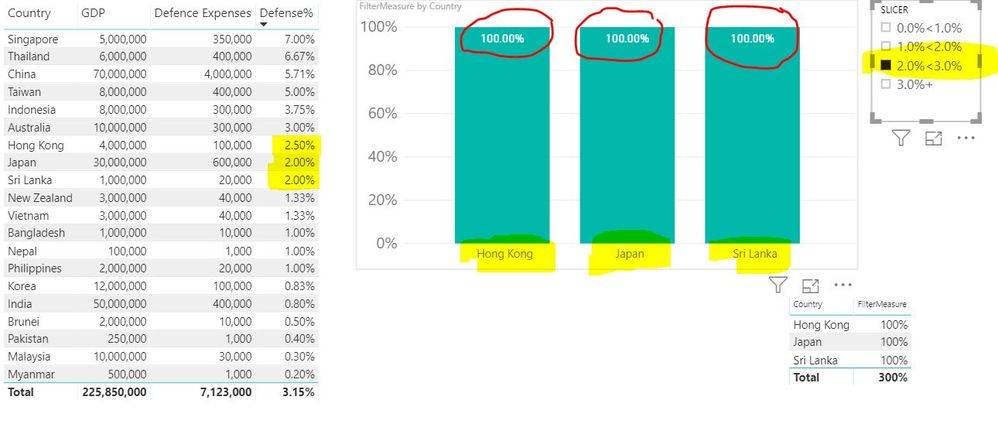Super User

[filterMeasure] is only meant to do just that, it filters the data based on your slicer selection, and returns the number of rows which satisfy the current filter context.

Since you have not posted any data or pbix-file, I have scraped together some data which resembles your data here:
GDP vs Military expenditure

Since I don't know how your data look like, you might have to consider the relationship between your tables. And also possibly count the 'Military expenditure'-table instead of the GDP-tableResolver II

This is brillaint,, i replicatre what you did and it works  ,,,,,, so great ,,, i see how u use visual filter, count row to amke this work...

@sturlaws wrote:

[filterMeasure] is only meant to do just that, it filters the data based on your slicer selection, and returns the number of rows which satisfy the current filter context.

Since you have not posted any data or pbix-file, I have scraped together some data which resembles your data here:
GDP vs Military expenditure

Since I don't know how your data look like, you might have to consider the relationship between your tables. And also possibly count the 'Military expenditure'-table instead of the GDP-tableHelper II

hi, what setting you use for visuals that needs to use the filterMeasure,

eg, if i add 1 visual with the exact axis, using your pbix file, it didnt filter like the rests of the visuals.

Must be some setting i missed out for the new visualHelper II

Hi @sturlaws ,

see the attached from the sample pbix, the visual i just added(with exact axis), doesnt filter correctly. (highlighted)

unless if i copy the visual directly, then the filter follows. That's what i meant earlier, there must be some step or setting i  missed to make it works automatically with the filterMeasure created.